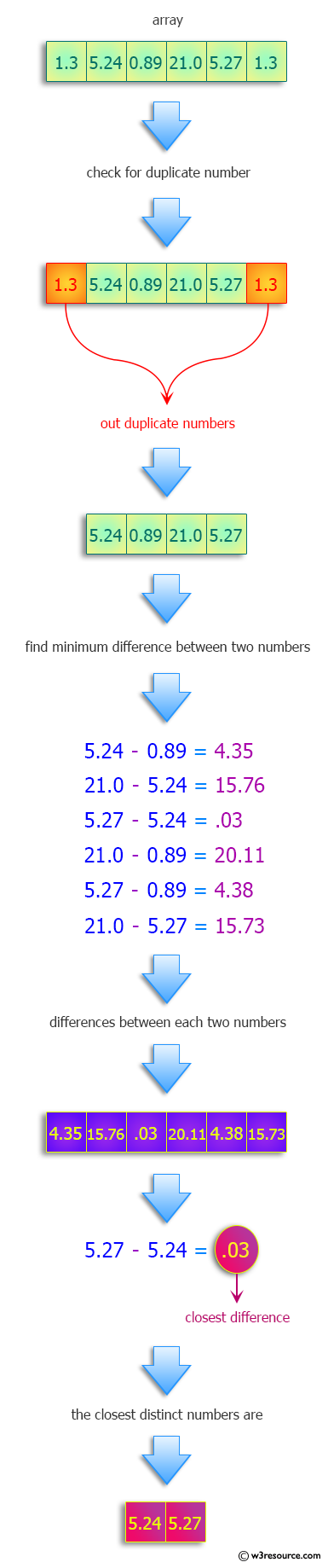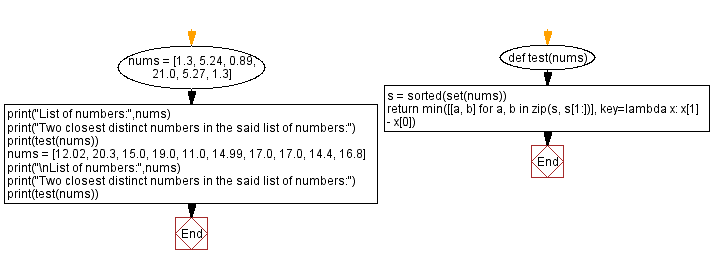﻿ Python: Find the two closest distinct numbers in a given a list of numbers - w3resource# Python: Find the two closest distinct numbers in a given a list of numbers

## Python Programming Puzzles: Exercise-78 with Solution

Write a Python program to find the two closest distinct numbers in a given a list of numbers.

```Input:
[1.3, 5.24, 0.89, 21.0, 5.27, 1.3]
Output:
[5.24, 5.27]

Input:
[12.02, 20.3, 15.0, 19.0, 11.0, 14.99, 17.0, 17.0, 14.4, 16.8]
Output:
[14.99, 15.0]
```

Pictorial Presentation:Sample Solution:

Python Code:

``````#License: https://bit.ly/3oLErEI

def test(nums):
s = sorted(set(nums))
return min([[a, b] for a, b in zip(s, s[1:])], key=lambda x: x - x)

nums = [1.3, 5.24, 0.89, 21.0, 5.27, 1.3]
print("List of numbers:",nums)
print("Two closest distinct numbers in the said list of numbers:")
print(test(nums))
nums = [12.02, 20.3, 15.0, 19.0, 11.0, 14.99, 17.0, 17.0, 14.4, 16.8]
print("\nList of numbers:",nums)
print("Two closest distinct numbers in the said list of numbers:")
print(test(nums))
``````

Sample Output:

```List of numbers: [1.3, 5.24, 0.89, 21.0, 5.27, 1.3]
Two closest distinct numbers in the said list of numbers:
[5.24, 5.27]

List of numbers: [12.02, 20.3, 15.0, 19.0, 11.0, 14.99, 17.0, 17.0, 14.4, 16.8]
Two closest distinct numbers in the said list of numbers:
[14.99, 15.0]
```

Flowchart:## Visualize Python code execution:

The following tool visualize what the computer is doing step-by-step as it executes the said program:

Python Code Editor :

Have another way to solve this solution? Contribute your code (and comments) through Disqus.

What is the difficulty level of this exercise?

Test your Programming skills with w3resource's quiz.

﻿

## Python: Tips of the Day

Clamps num within the inclusive range specified by the boundary values x and y:

Example:

```def tips_clamp_num(num,x,y):
return max(min(num, max(x, y)), min(x, y))
print(tips_clamp_num(2, 4, 6))
print(tips_clamp_num(1, -1, -6))
```

Output:

```4
-1
```